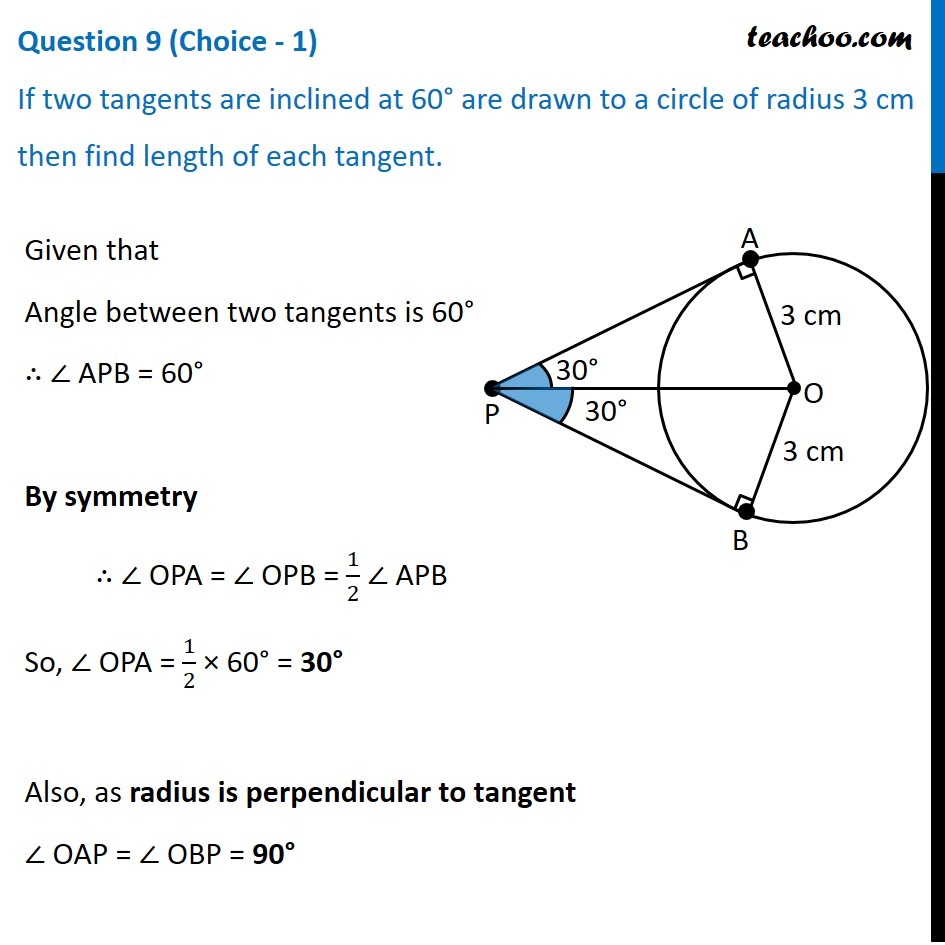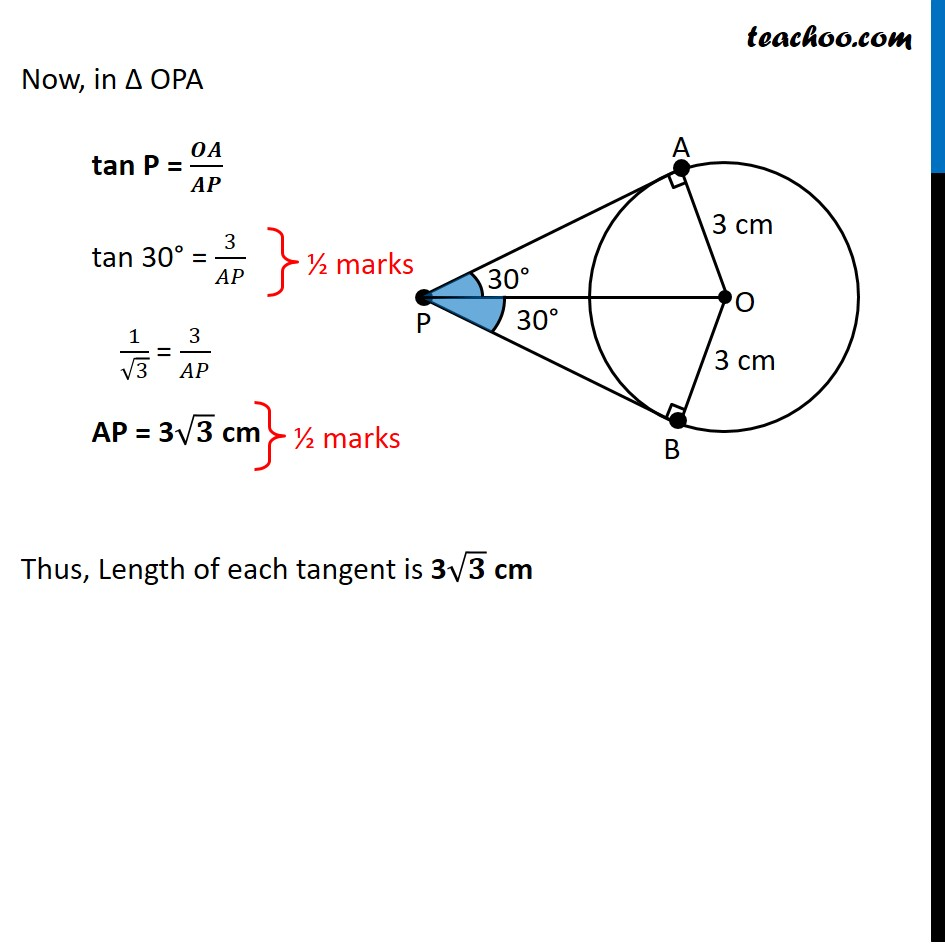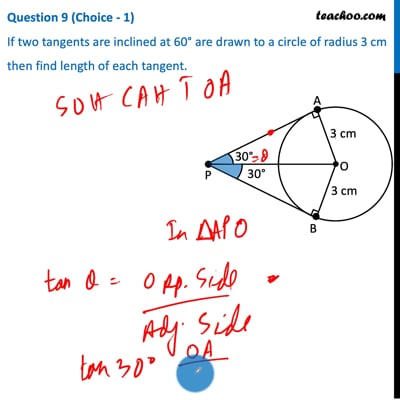CBSE Class 10 Sample Paper for 2021 Boards - Maths Standard

Class 10
Solutions of Sample Papers for Class 10 Boards

## If two tangents are inclined at 60° are drawn to a circle of radius 3 cm then find length of each tangent.This video is only available for Teachoo black users

Introducing your new favourite teacher - Teachoo Black, at only ₹83 per month

### Transcript

Question 9 (Choice - 1) If two tangents are inclined at 60° are drawn to a circle of radius 3 cm then find length of each tangent. Given that Angle between two tangents is 60° ∴ ∠ APB = 60° By symmetry ∴ ∠ OPA = ∠ OPB = 1/2 ∠ APB So, ∠ OPA = 1/2 × 60° = 30° Also, as radius is perpendicular to tangent ∠ OAP = ∠ OBP = 90° Now, in Δ OPA tan P = 𝑶𝑨/𝑨𝑷 tan 30° = 3/𝐴𝑃 1/√3 = 3/𝐴𝑃 AP = 3√𝟑 cm Thus, Length of each tangent is 3√𝟑 cm